## Introduction

Initial Cu L3-edge resonant inelastic X-ray scattering (RIXS) measurements on undoped and weakly underdoped cuprates1,2 complemented earlier neutron and Raman scattering experiments, seeming to favour a spin-fluctuation scenario as a viable explanation of superconductivity3. However, more recent RIXS measurements on overdoped cuprates3,4,5,6 have shown persistent high-energy spin excitations to very high doping levels where superconductivity disappears. In contrast neutron and Raman measurements display an absence of robust spin excitations in the overdoped regime7,8; this conflict undermines an understanding of unconventional superconductivity in the cuprates, making an investigation of how spin excitations manifest in the RIXS cross-section a crucial component to its resolution.

In this letter, we reconcile these seemingly incompatible experimental results by computing Cu L3-edge RIXS spectra using exact diagonalization (ED), capable of reproducing major experimental features. We demonstrate with light polarization analysis that the RIXS cross-section in a crossed-polarization geometry can be interpreted simply in terms of the spin dynamical structure factor S(q, ω), which enables a comparison between different scattering experiments. Utilizing determinant quantum Monte Carlo (DQMC), we study in detail the momentum and doping dependence of S(q, ω), finding strong changes near both (π, π) and (0, 0) with relatively insensitive antiferromagnetic zone boundary (AFZB) paramagnons upon hole doping. Moreover, with electron doping these same AFZB paramagnons harden significantly, which has recently been confirmed experimentally. Underlying this observed behaviour is a framework of local spin exchange, which remains robust even with significant doping away from the parent antiferromagnet. In contrast, our calculations show a sensitive evolution of low-energy paramagnons near (0, 0) and (π, π), which give evidence for the predominance of ferromagnetic correlations. These results highlight the importance of spectral weight and dispersion at low energies in establishing a relevant energy scale and strength of spin fluctuations for pairing rather than higher-energy AFZB paramagnons.

## Results

### The relationship between RIXS and S(q, ω)

RIXS is a resonant technique and its sensitivity to magnetic excitations arises as a result of core-level spin-orbit interactions in the intermediate state (see Fig. 1a). Although in Mott insulators it has been shown that the RIXS cross-section can be approximated by S(q, ω) when the charge excitations are gapped, it is not clear whether the same approximation carries over to doped systems where the ground state is no longer that of a Mott insulator with commensurate filling9,10.Figure 1: Similarities between RIXS and the spin dynamical structure factor S(q, ω).

To answer this question, we numerically evaluate the RIXS cross-section as a function of momentum (Fig. 1c) directly from the Kramers-Heisenberg formula11,12 using small cluster ED of an effective single-band Hubbard Hamiltonian (including both nearest t and next-nearest neighbour hopping t′ and on-site Coulomb repulsion U) at various electron concentrations n; details are given in the Methods section. Figure 1c displays the RIXS spectra calculated for the experimental geometry discussed in ref. 3. The RIXS spectra, even without outgoing polarization discrimination, agree well with S(q, ω) for different electron concentrations at the chosen momentum space points accessible on the same finite size clusters for each calculation. This is particularly true at half-filling where the charge gap ensures that only spin excitations can be visible in the given energy range. The main differences occur in the doped systems at higher energy (close to 2t), which are of less interest for our spin analysis. The important result shown in Fig. 1c concerns the suppression of these higher energy peaks in the cross-polarized geometry (see Fig. 1c π−σ RIXS) which leads to a significant improvement in the comparison between the RIXS cross-section and S(q, ω). This indicates that Cu L3-edge RIXS with crossed polarizations (a four-particle correlator) provides access to the spin excitation spectrum encoded in S(q, ω) (a two-particle correlator) for doped as well as undoped cuprates. (To further confirm this agreement between RIXS and S(q, ω) for more momentum points, we manually adjusted the Cu L-edge energy to be 1.8*930 eV=1674, eV so that momentum points up to (π, π) can be reached. For more details see Supplementary Note 1.)

### The momentum and doping dependence of S(q, ω)

Having established a relationship between RIXS and S(q, ω), we focus now on the momentum and doping dependence of S(q, ω) for the single-band Hubbard model. Here we employ the numerically exact DQMC method (see Methods) with maximum entropy analytic continuation on larger lattices with fine control of the electron concentration through the chemical potential. As shown in Fig. 2 the DQMC calculations qualitatively reproduce both the momentum and doping evolution of the RIXS measurements found in refs 1, 2, 3, 4, 5, 6. A comparison between the intensity and dispersion of low-energy magnetic excitations near (0, 0) and (π, π) shows a transition from antiferromagnetic to ferromagnetic spin correlations with increasing doping. [In an antiferromagnetic system, the dynamical spin structure factors show gapless excitations at both (0, 0) and (π, π), with strong intensity around (π, π). In a ferromagnetic system, the dynamical spin structure factors show strong intensity with gapless excitations at (0, 0) and much weaker intensity with gapped excitations when approaching (π, π)]. However, the spectra of higher-energy AFZB paramagnons show relatively little change with hole doping other than a general decrease of intensity, suggesting that spin excitations do not soften even in the heavily overdoped regime. For electron doping, AFZB paramagnons surprisingly harden by 50% at 15% doping that has been observed recently in the prototypical electron-doped cuprate Nd2−xCexCuO4 (ref. 13). For additional analysis and discussion, see Supplementary Discussion.

### Theory to understand AFZB paramagnons

The behaviour of these AFZB paramagnons stands in stark contrast to naive expectations of spin softening with either hole or electron doping: (i) long-range AF order collapses quickly upon doping with a small (intermediate) concentration of holes (electrons), and one would expect spin excitations to soften accordingly14,15; (ii) short-range AF correlations are further weakened due to a dilution of AF bonds (see Fig. 3a) in the locally static spin picture. Indeed, the nearest neighbour spin-spin correlations from the DQMC calculations with electron doping decrease in a manner surprisingly well described by a locally static spin picture where the doped electrons are immobile, as shown in Fig. 3b.Figure 2: The spin dynamical structure factor S(q, ω) calculated using DQMC for the Hubbard model.

We can address these points by considering the role of three-site exchange16, which lowers the system energy when both doped carriers and AF correlations are present (see Fig. 3a). A local spin flip in an otherwise AF background produces a ferromagnetic alignment of nearest neighbour spins, which costs additional energy (of the same order as the spin exchange J=4t2/U) by suppressing hole- (or double-occupancy-) delocalization represented by the three-site terms. In fact, if we consider only the spin exchange contributions, the combined energy of a single-spin flip in the doped system (breaking both spin exchange and three-site bonds) is larger than that of the undoped system by ~J/4 (see Fig. 3a). This hardening has been observed in ED calculations of S(q, ω) for the HHubbard and Ht−J+H3s Hamiltonians (see Methods) as shown in Fig. 3c upon electron doping.

The situation is more subtle with hole doping, because this ‘locally static model’ no longer completely applies as seen in Fig. 3b. The negative next-nearest neighbour hopping t′ (positive for electron doping) promotes magnetic sublattice mixing and a much larger destruction of the AF correlations17. With hole doping the trend observed in RIXS is fully recovered only in the Hubbard model, as shown in Fig. 2, implying that higher order processes absent in tJ-type models become crucial in quantitatively reproducing the spin wave dispersion18.

## Discussion

How do these results reconcile the seemingly contradictory observations between RIXS, neutron and Raman scattering? First, inelastic neutron scattering probes spin excitations particularly well around (π, π) momentum transfer, showing a vanishing spectral weight in the regime of large hole doping p0.3 (refs 7, 19). This behaviour is also visible in the numerical results presented in Fig. 2a, which suggest that the impact of doping on the intensity and dispersion of excitations near (0, 0) and (π, π) is not symmetric. The decreasing correlation length with doping, evidenced by the spin gap at (π, π) and the weak dispersion towards (π/2, π/2), thus impacts these momentum points more strongly than the AFZB paramagnons, in accordance with a locally static picture. Second, Raman scattering8,20,21 shows a softening of the so-called bimagnon (double spin-flip or two-magnon) response upon both hole and electron doping. This trend has been reproduced by our ED calculations of the B1g Raman response shown in Fig. 4 (see Methods for the calculation details and the verification of bimagnon peaks). Strong magnon-magnon interactions reduce the bimagnon Raman peak energy from twice that of the single-magnon bandwidth as determined by AFZB magnons and quickly reduce the overall intensity. Taken as a whole, our results provide a qualitative, and in some cases quantitative, agreement with the salient experimental features of neutron scattering, Raman and RIXS measurements, suggesting that coherent propagating spin waves quickly disappear with the destruction of long-range AF order upon doping, while short-range, single spin-flip processes can survive to high doping levels as reflected in the evolution of S(q, ω).

Full polarization control will allow RIXS to become an effective tool for directly observing spin dynamics along the AFZB, particularly noting the electron/hole doping differences. Together with the dome-shaped superconducting phase diagram, these results imply that AFZB spin fluctuations might play a relatively minor role in the pairing mechanism, consistent with established experimental and numerical observations22,23,24. This calls into question a simple view of pairing that emphasizes only the spin exchange energy scale J. However, we suggest that a definitive resolution to this issue would come from future RIXS experiments along the BZ diagonal (out to (π/2, π/2)) to illuminate the evolution from antiferro- to ferro-magnetic correlations, compare with neutron scattering results and ultimately shed additional light on the intriguing mystery of cuprate high-temperature superconductivity.

## Methods

### Numerical techniques

We use exact diagonalization (ED) to evaluate the RIXS cross-section from the Kramers–Heisenberg formula11, spin dynamical structure factor S(q, ω) and Raman scattering cross-section25 on small clusters with periodic boundary conditions. We employ a 12-site Betts cluster26 in evaluating the RIXS cross-section and S(q, ω) shown in Fig. 1. The Raman scattering response shown in Fig. 4 has been evaluated on 16- and 18-site square (or diamond-shaped) clusters, and the 18-site cluster was employed to evaluate S(q, ω) for HHubbard, Ht−J and Ht−J+H3s shown in Fig. 3. The ED calculations for HHubbard are performed with the Parallel ARnoldi PACKage (PARPACK) and the cross-sections obtained by use of the biconjugate gradient stabilized method and continued fraction expansion12. The ED calculations on Ht−J and Ht−J+H3s models are performed using the Lanczos algorithm. Finite temperature DQMC simulations27,28 were performed on HHubbard to obtain the imaginary time spin-spin correlation function from which the real frequency response function S(q, ω) was obtained by analytic continuation using the maximum entropy method (MEM)29,30. These simulations were performed on 8 × 8 square lattice clusters with periodic boundary conditions at an inverse temperature β=3/t for the same Hubbard Hamiltonian parameter values utilized in the ED studies. For this set of parameters, the DQMC method exhibits a significant fermion sign problem31 over the entire doping range, which we address in the MEM30 (see Supplementary Methods and Supplementary Fig. 6). MEM requires the use of a model function for determining an entropic prior in the analytic continuation routine. We utilize a Lorentzian model whose peak as a function of q is determined from a simple spin wave dispersion at small q out to the AFZB; however, beyond the AFZB the model assumes no softening as expected for long-range antiferromagnetism with the top of the magnon band set by approximations for the spin exchange J and an assumed reduction of the spin moment by quantum fluctuations. While some quantitative changes occur with significant changes to these default models, we have checked that the qualitative behaviour remains robust. The MEM routine returns the real frequency spin susceptibility from which S(q, ω) is obtained from the fluctuation-dissipation theorem. More details about the models and numerical algorithms can be found in the following Methods and Supplementary Methods.

### RIXS

The Cu L3-edge RIXS cross-section is calculated using the Kramers–Heisenberg formula11 for the single-band Hubbard model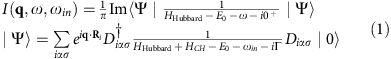in which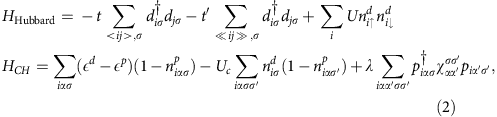where q is the momentum transfer; ωin and ω=ωinωout are the incident photon energy (in our study the Cu L3-edge) and photon energy transfer, respectively; E0 is the ground state energy of the system in the absence of a core-hole; |0› is the ground state wave function;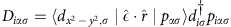(and h.c.) dictates the dipole transition process from Cu 2p to the 3d level (or from Cu 3d to 2p), with the X-ray polarization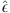either π or σ (the polarization vector parallel or perpendicular to the scattering plane); and Γ is the inverse core-hole lifetime (see Supplementary Note 2). In HHubbard, <…> and … represent a sum over the nearest and next-nearest neighbour sites, respectively. The Hamiltonian for the intermediate state also involves the on-site energyfor creating a 2p core hole, Coulomb interaction Uc induced by the core-hole and spin-orbit coupling λ, all denoted as in HCH.represents the spin-orbital coupling coefficients. The angle between the incident and the scattered photon propagation vectors is set to be 50°. The parameters used in the RIXS calculation are t=0.4 eV, U=8t=3.2 eV, t′=−0.3t=−0.12 eV,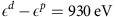, Uc=−4t=−1.6 eV, λ=13 eV and Γ=1t=0.4 eV (refs 32, 33). RIXS spectra at half-filling are taken only at the Cu L3 resonance, and upon doping at the resonance closest to the half-filling Cu L3-edge resonant energy. The RIXS results were obtained for a Lorentzian broadening with half width at half maximum (HWHM)=0.01 eV (0.025t) and a Gaussian broadening with HWHM=0.047 eV (0.118t) on the energy transfer. The spin dynamical structure factor S(q, ω), discussed in the next section, for HHubbard was calculated using the same parameters to make comparison to our RIXS results.

### Spin dynamical structure factor

The spin dynamical structure factor is defined as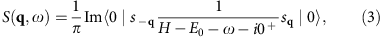where we have studied the HHubbard, Ht−J and Ht−J+H3s Hamiltonians: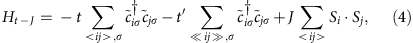E0 is the corresponding ground state energy of the model Hamiltonian;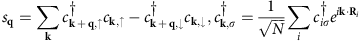for HHubbard;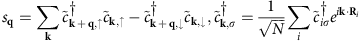, for Ht−J and H3s;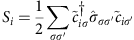; and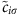is restricted in the subspace without double occupancy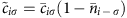and, in which the operator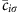annihilates a dressed electron whose hopping conserves the number of effective doubly occupied sites34. To explore the similarities and differences between HHubbard and Ht−J (with and without H3s), we calculate S(q, ω) on the three model Hamiltonians with the parameters J=0.4t, t′=−0.25t and U=10t (corresponding to J=0.4t by the relation J=4t2/U).

### Locally static model

In the ‘locally static model’ (see Fig. 3b) it is assumed that the holes destroy the short-range spin-spin correlations solely by the effect of ‘static’ doping, that is, by removing the spins and thus cutting spin bonds. In this case, the nearest neighbour spin-spin correlation can be calculated in the following way:where 〈S0S1› is the abbreviation of 〈0|SiSj|0› for two neighbouring sites i and j, and p is the concentration of either doped holes (P=1−n) or doped electrons (P=n−1).

### Raman scattering

We calculate the Raman scattering cross-section in the B1g channel using the non-resonant response function for HHubbard25: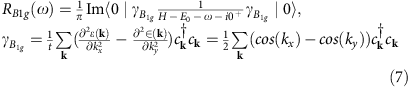in which ?(k)=−2t(coskx+cosky)−4tcoskxcosky is the bare band dispersion, with parameters U=8t and t′=−0.3t. t is taken as 0.4 eV to make comparison with experimental data.

This two-particle response also has been studied recently in cluster dynamical mean-field theory35 showing that, if calculated fully gauge invariantly, the nonresonant Raman B1g response shows the presence of a strong bimagnon peak at half filling. Nevertheless, the Raman spectrum calculated using this method for doped systems is sensitive to both charge and spin excitations in the low-energy regime. Our identification of the bimagnon excitations in the Raman spectra relies primarily on the qualitative evolution of the peaks in agreement with experimental observations20. We note that all of the excitations visible in our Raman spectra correspond to ΔS=0 transitions that have B1g symmetry. At half-filling, the energy of the excitation to which we assign bimagnon character lies within the charge gap, which makes the bimagnon assignment clear. Upon either hole or electron doping, we expect to develop charge excitations in the Raman response at low energy and for the two-magnon response to soften and decrease in intensity. Our assignment corresponds to an upper bound for the bimagnon energy scale with doping where the additional structure at low energies signals either charge excitations or a substantial broadening of the bimagnon excitations now represented by multiple features in the ED result (as discussed in connection with comparisons between DQMC and ED results). However, the energy scale clearly softens and, more importantly, the intensity drops (significantly) in agreement with the experimental observations where the bimagnon ‘peak’ becomes nearly impossible to distinguish from the charge background almost immediately upon crossing the AFM phase boundary.